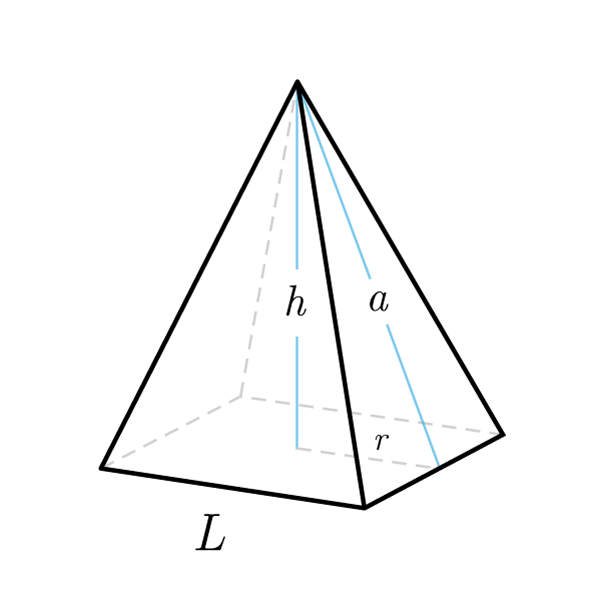# Pyramid

 Edge $$S$$ Radius $$r$$ Height $$h$$ Apothem $$a$$
$$V = \frac{A_{base} \times h}{3}$$
Volume
$$A_{base} = \frac{3V}{h}$$
Base area
$$h = \frac{3V}{A_{base}}$$
Height
$$S_{tot} = A_{base} + S_{lat}$$
Total surface
$$S_{lat} = S_{tot} - A_{base}$$
Lateral surface
$$A_{base} = S_{tot} - S_{lat}$$
Base area
Right Pyramid
$$S_{lat} = \frac{2p \times a}{2}$$
Lateral surface
$$2p = \frac{2 \times S_{lat}}{a}$$
Base perimeter
$$a = \frac{2 \times S_{lat}}{2p}$$
Apothem
$$r = \frac{2 \times A_{base}}{2p}$$
$$2p = \frac{2 \times A_{base}}{r}$$
Base perimeter
$$A_{base} = \frac{2p \times r}{2}$$
Base area
By using the Pythagoras' Theorem
$$a = \sqrt{{h}^2 + {r}^2}$$
Apothem
$$r = \sqrt{{a}^2 - {h}^2}$$
$$h = \sqrt{{a}^2 - {r}^2}$$
Height
$$A_{base} = {S}^2$$
Base area
$$S = \sqrt{A_{base}}$$
Base Edge
$$2p = S \times 4$$
Base perimeter
$$S = \frac{2p}{4}$$
Base Edge
$$r = \frac{S}{2}$$
$$S = 2 r$$
Base Edge

## Definition

A pyramid is a polyhedron formed by a polygonal face, called base, and a point external to the base, called vertex.

## Properties

1. In a pyramid there is a base face and lateral faces
2. The lateral face of a pyramid are isosceles triangles congruent to each other
3. Height: perpendicular segment that connects the vertex of the pyramid with the plane that contains the base
4. Apothem: in the regular pyramid, segment that joins the vertex with the midpoint of the base edge. It coincides with the height of one of the triangles that make up the lateral faces

## Other definitions

• A right pyramid is a pyramid in which the base polygon is inscribed in a circle, and the height joins the vertex with the center of the inscribed circle
• An oblique pyramid is any pyramid that is not a right pyramid
• A regular pyramid is a pyramid having a regular polygon as its base## Pyramid Formulas

Data Formula
Volume V = (Abase × h) / 3
Base area Abase = (3V) / h
Height h = (3V) / Abase
Total surface Stot = Abase + Slat
Lateral surface Slat = Stot - Abase
Base area Abase = Stot - Stot

## Right Pyramid Formulas

Data Formula
Lateral surface Stot = (2p × a) / 2
Base perimeter 2p = (2 × Slat) / a
Apothem a = (2 × Slat) / 2p
Inscribed radius r = (2 × Abase) / 2p
Base perimeter 2p = (2 × Abase) / r
Base area Abase = (2p × r) / 2
Apothem a = √(h2 + r2)
Base radius r = √(a2 - h2)
Height h = √(a2 - r2)